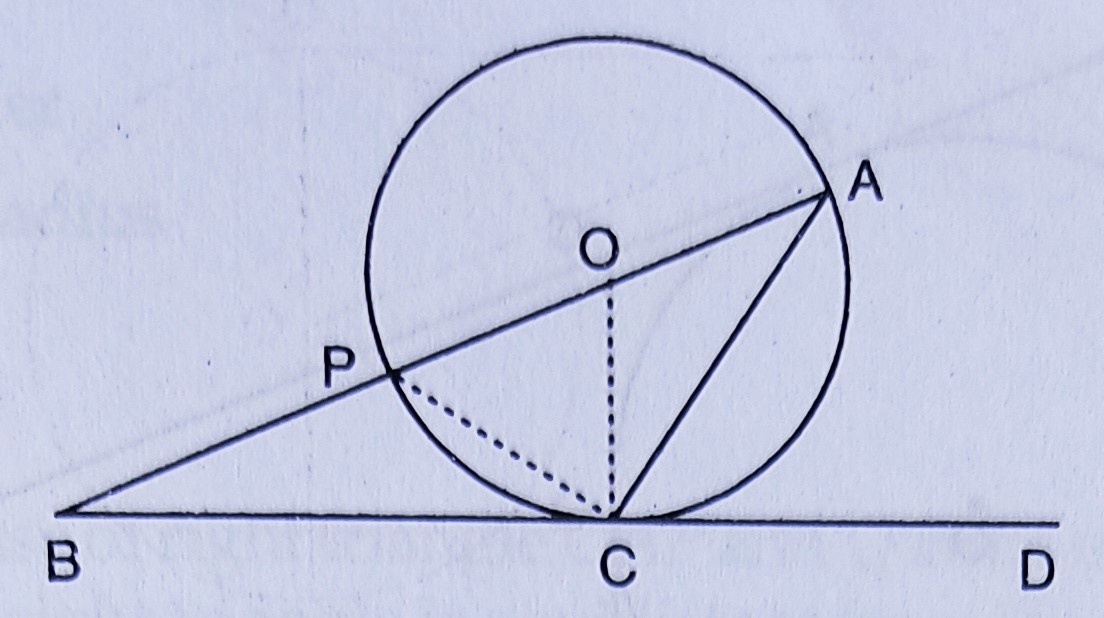"
">

# In the figure, $O$ is the centre of the circle and $B C D$ is tangent to it at $C$. Prove that $\angle B A C+\angle A C D=90^{\circ}$."

Given:

In the figure, $O$ is the centre of the circle and $B C D$ is tangent to it at $C$.

To do:
We have to prove that $\angle B A C+\angle A C D=90^{\circ}$.

Solution:

From the figure,

$\angle ACD = \angle CPA$     (Angles in the alternate segment are equal)

In $\triangle ACP$,

$\angle ACP = 90^o$    (Angle in a semicircle)

$\angle PAC + \angle CPA = 90^o$

$\angle BAC + \angle ACD = 90^o$     ($ACD = ∠CPA$)

Hence proved.

Updated on: 10-Oct-2022

26 Views